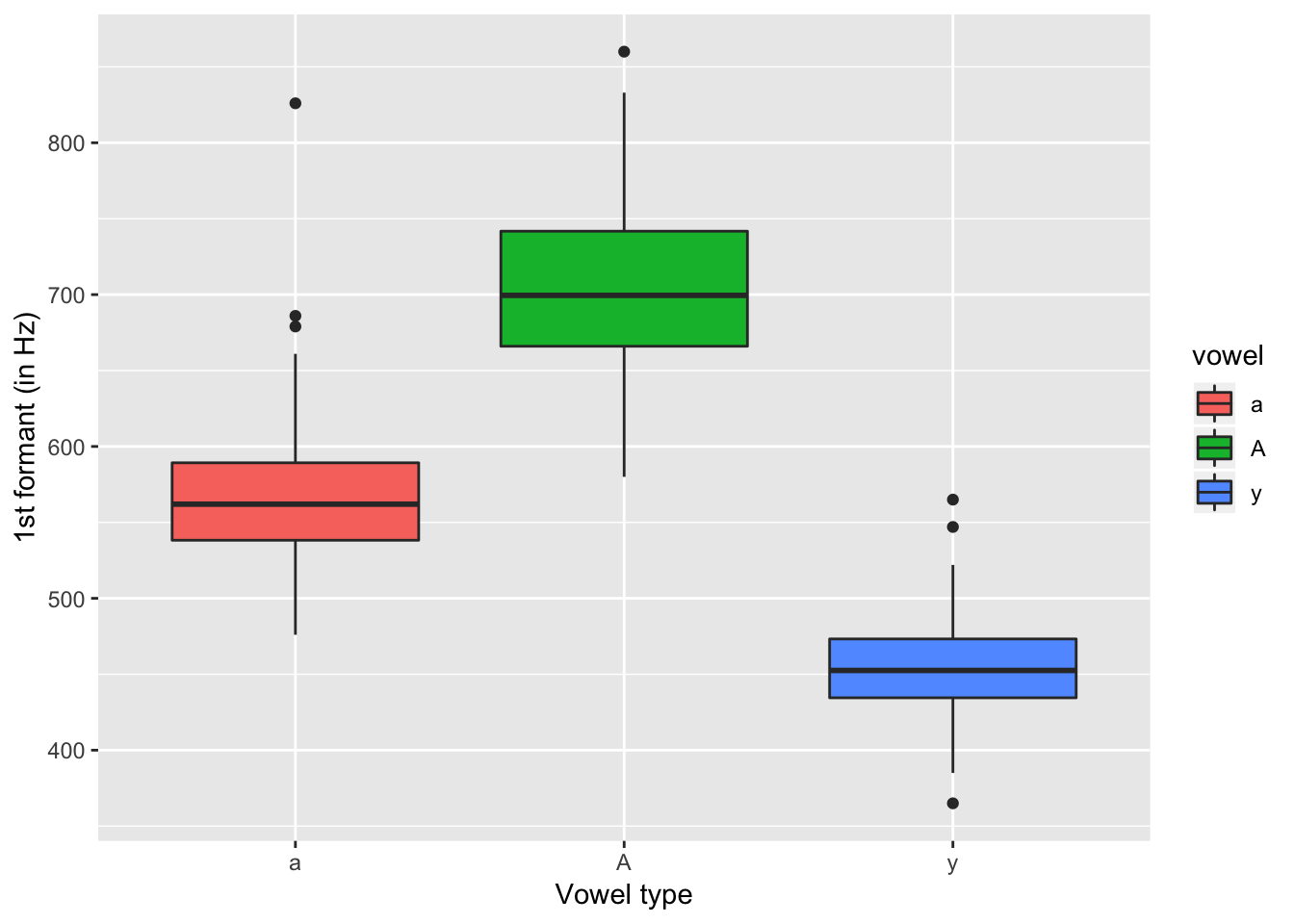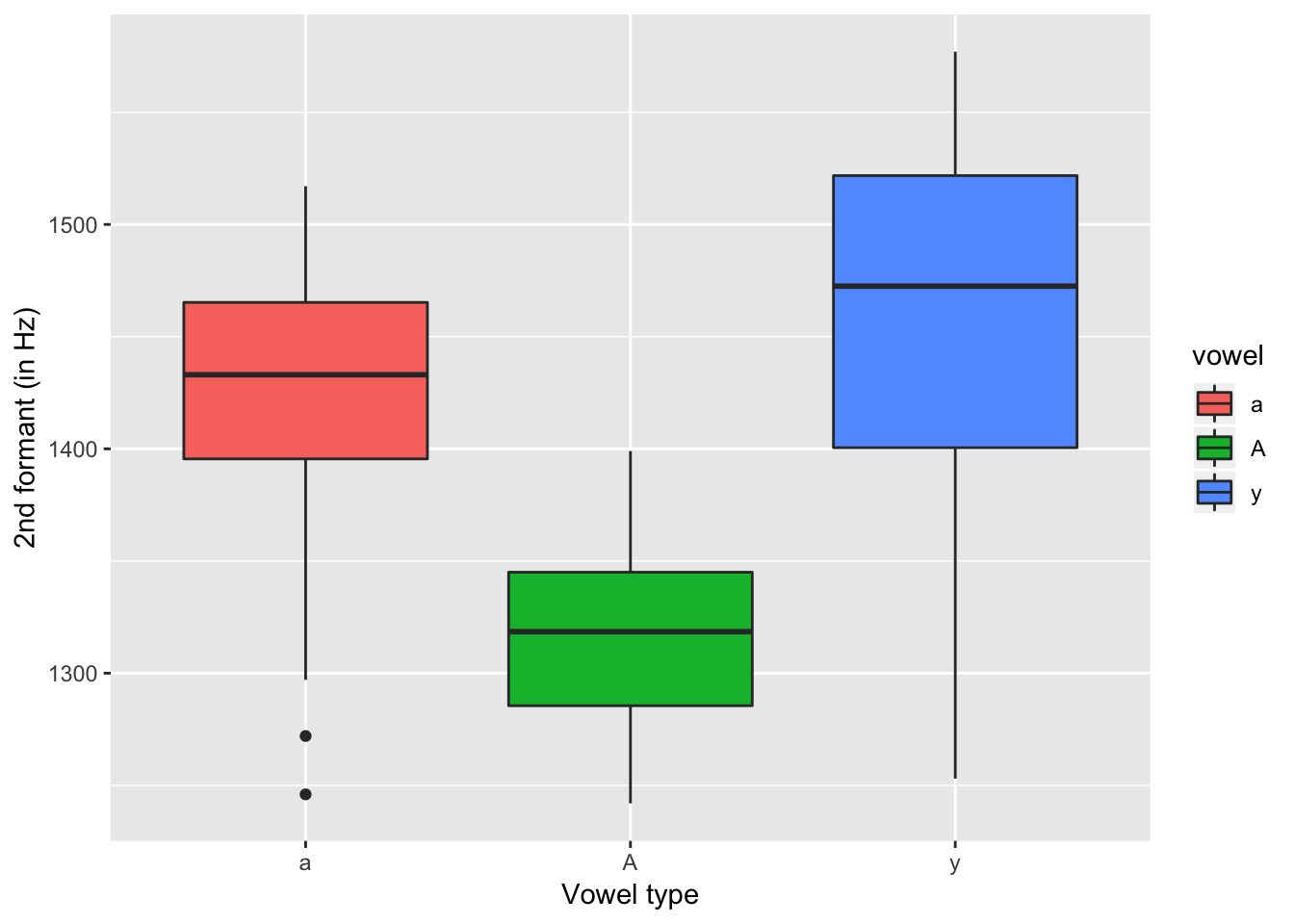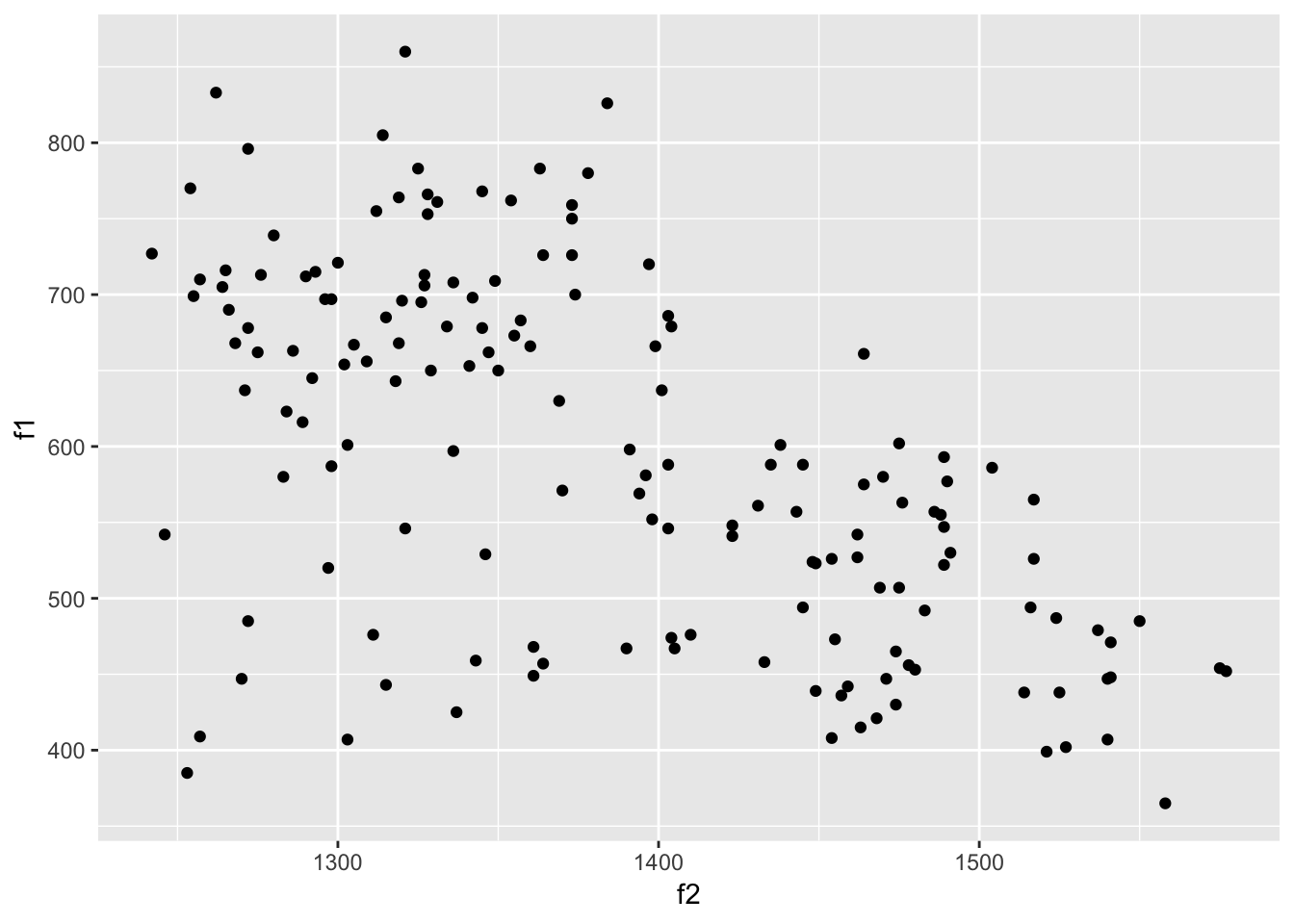## Linear mixed-effects models

Based on this Lab11.

At this seminar we will work with the data set `ReductionRussian.txt`. Pavel Duryagin ran an experiment on perception of vowel reduction in Russian language. The data set includes the following variables:

• `time1`: reaction time 1;
• `time2`: reaction time 2;
• `duration`: duration of the vowel in the stimuly (in milliseconds, ms);
• `f1`: the first formant (if don’t know about formants, see here);
• `f2`: the second formant;
• `f3`: the third formant;
• `vowel`: vowel type.

Vowel classified according the 3-fold classification (`A` - a under stress, `a` - a/o as in the first syllable before the stressed one, `y` (stands for shva) - a/o as in the second etc. syllable before the stressed one or after the stressed syllable, cf. g[y]g[a]t[A]l[y], gogotala, ‘guffawed’).

Our goal for today is to understand how the first formant depends on the values of the second formant and whether this relationship is different for different types of vowels.

Let us load this data set from the txt-file using `read.table()` function. Please, note that we should add the option `header=TRUE` to tell R that the first row should be read as a row with column names.

``sh <- read.table("https://raw.githubusercontent.com/LingData2019/LingData/master/data/duryagin_ReductionRussian.txt", header=TRUE)``

Look at the summary of our data and make sure all variables have correct types:

``summary(sh)``
``````##      time1            duration           time2               f2
##  Min.   :  3.659   Min.   :0.01455   Min.   :  3.721   Min.   :1242
##  1st Qu.: 39.082   1st Qu.:0.04388   1st Qu.: 39.109   1st Qu.:1315
##  Median : 88.232   Median :0.06336   Median : 88.266   Median :1372
##  Mean   : 93.227   Mean   :0.07272   Mean   : 93.261   Mean   :1386
##  3rd Qu.:143.149   3rd Qu.:0.09769   3rd Qu.:143.191   3rd Qu.:1462
##  Max.   :191.228   Max.   :0.16493   Max.   :191.244   Max.   :1577
##        f1              f3       vowel
##  Min.   :365.0   Min.   :1718   a:44
##  1st Qu.:483.5   1st Qu.:2162   A:68
##  Median :587.5   Median :2216   y:48
##  Mean   :592.8   Mean   :2220
##  3rd Qu.:695.2   3rd Qu.:2302
##  Max.   :860.0   Max.   :2426``````

As later we will work with mixed-effects models, it is important to understand how many rows with missing values our data frame has (mixed-effects models work correctly when the share of NA’s is small).

Let’s count rows with missing values:

``````# ! - negation of complete.cases()
sum(!complete.cases(sh))``````
``##  0``

As we see, no rows with missing values are detected, we can go on.

In our data we have three groups of vowels (see above). Let’s look at the summary statistics by groups:

``````library(tidyverse)

sh %>% group_by(vowel) %>% summarise(n = n(),
mean_f1 = mean(f1),
mean_f2 = mean(f2))``````
``````## # A tibble: 3 x 4
##   vowel     n mean_f1 mean_f2
##   <fct> <int>   <dbl>   <dbl>
## 1 a        44    573.   1421.
## 2 A        68    704.   1316.
## 3 y        48    454.   1452.``````

As we can see, the groups are approximately of the same size (balanced), and the mean values of the first formant and of the second formant differ by groups. Now we can visualize the distribution of formants by groups using `ggplot2`.

``````ggplot(data = sh, aes(x = vowel, y = f1, fill = vowel)) +
geom_boxplot() +
labs(x = "Vowel type", y = "1st formant (in Hz)")````````````ggplot(data = sh, aes(x = vowel, y = f2, fill = vowel)) +
geom_boxplot() +
labs(x = "Vowel type", y = "2nd formant (in Hz)")``````Again we can see the differences by groups: the median values of `f1` and `f2` are different, the range of values is also different. Now we visualize the relationship between `f1` and `f2`:

``ggplot(data = sh, aes(x = f2, y = f1)) + geom_point()``This scatter plot is interesting. On the one hand, the relationship between `f1` and `f2` is negative: the higher are the values of `f2`, the lower the values of `f1` are. On the other hand, if we take a closer look, we will see that there are different groups of points, and the relationship between `f1` and `f2` can be different as well. Now let us add grouping to this graph:

``ggplot(data = sh, aes(x = f2, y = f1, color = vowel)) + geom_point()``